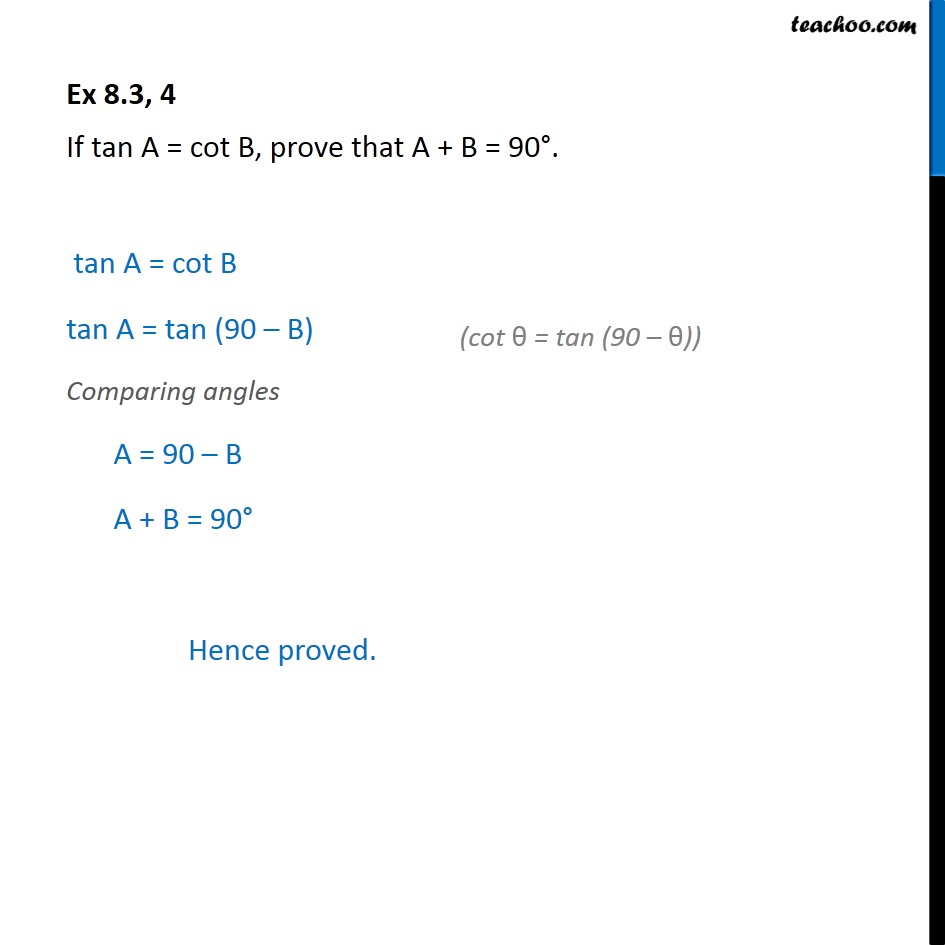Sin 90 - θ, cos 90 - θ formula

Chapter 8 Class 10 Introduction to Trignometry
Serial order wiseLearn in your speed, with individual attention - Teachoo Maths 1-on-1 Class

### Transcript

Question 4 If tan A = cot B, prove that A + B = 90 . Given tan A = cot B To solve this we should know relation between tan and cot Now, We know that cot θ = tan (90 - θ) Putting value of cot B tan A = tan (90 - B) Comparing angles A = 90 - B A + B = 90 Hence proved.